# 4. 嵌入式操作系统复习

## 概念

• 实时性（中断响应时间、任务切换时间等）
• 尺寸（可裁剪性 ）
• 可扩展性（内核、中间件）

• 开放性、可伸缩性的体系结构。
• 强实时性。
• 统一的接口。
• 操作方便、简单、提供友好的图形GUI。
• 提供强大的网络功能。
• 强稳定性，弱交互性。
• 固化代码。
• 更好的硬件适应性，也就是良好的移植性。

• 用冯•诺依曼（Von Neumann）结构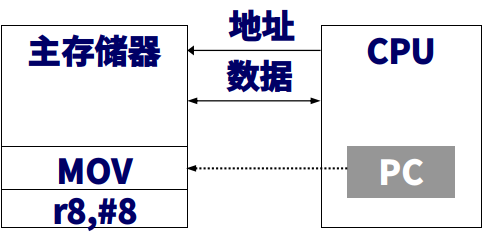• 哈佛（Harvard）结构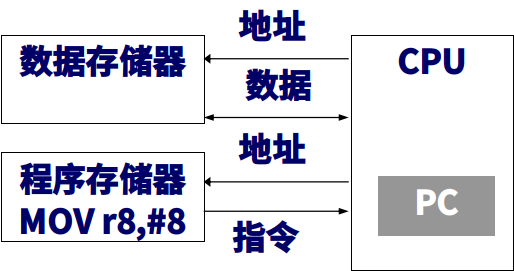• 体系结构是操作系统的基础，它定义了硬件与软件的界限、内核与操作系统其它组件（文件、网络、GUI等）的组织关系、系统与应用的接口。
• 体系结构是确保系统的性能、可靠性、灵活性、可移植性、可扩展性的关键，就好比房子的梁架，只有梁架搭牢固了才提得上房子的质量，再做一些锦上添花的工作才有意义。
• 目前操作系统的体系结构可分为：单块结构、层次结构和客户/服务器（微内核）结构。

• 分层和模块化结合的结构将操作系统分为硬件无关层、硬件抽象层和硬件相关层，每层再划分功能模块。
• 采用微内核结构，则可利用其可伸缩的特点适应硬件的发展，便于扩展。
ucos操作系统是一个什么样的系统
μC/OS-II是近年来国内比较流行的一种嵌入式操作系统,是一种专门为嵌入式设备设计的，基于优先级的可抢先式的硬实时EOS内核。

• 实时系统指系统的计算正确性不仅取决于计算的逻辑正确性，还取决于产生结果的时间。如果未满足系统的时间约束，则认为系统失效。
• 一个实时操作系统面对变化的负载时必须确定性地保证满足时间要求

• 硬实时系统指系统要有确保的最坏情况下的服务时间，即对于事件的响应时间的截止期限是无论如何都必须得到满足。
• 软实时系统就是那些从统计的角度来说，一个任务能够得到有确保的处理时间，到达系统的事件也能够在截止期限到来之前得到处理，但违反截止期限并不会带来致命的错误。

• 静态优先级：任务的优先级被确定后，在系统运行过程中将不再发生变化；
• 动态优先级：系统运行过程中，任务的优先级是可以动态变化的。

• 相对截止时间为任务的绝对截止时间减去 任务的就绪时间。
• 强截止时间的任务即为关键任务，如果截止时间不能得到满足，就会出现严重的后果。
• 关键任务的实时系统又被称为强实时（hard real-time）系统，否则称为弱实时（soft real-time）系统。
uCos的五个状态？
• 运行态(running)：已经获取CPU使用权并运行的任务
• 等待态(waiting)：暂时让出CPU使用权，等待某一事件触发（信号量、事件标志，信号队列）
• 中断服务态(ISR):正在执行的任务被中断任务打断，UCOS执行中断任务什么是系统空闲任务。
• 当没有其他任务准备好运行时执行该命令。
• 空闲任务始终设置为最低优先级。
• 空闲任务永远不能被应用程序软件删除。
• 空闲任务由操作系统创建。

• 便于应用能够向系统中添加一些关于任务的附加操作
• 为应用提供在系统运行的关键点上进行干预的手段

• 单等待队列
• 资源对应的事件发生时，内核需要扫描整个等待队列，搜索等待该资源的任务，并按照一定的策略选取任务，把任务的任务控制块放置到就绪队列。
• 如果系统的资源和任务比较多，搜索等待该资源的任务所需要的时间就比较长，会影响整个系统的实时性能。
• 多等待队列
• 资源对应的事件发生时，能够在较短的时间内确立等待该资源的任务等待队列。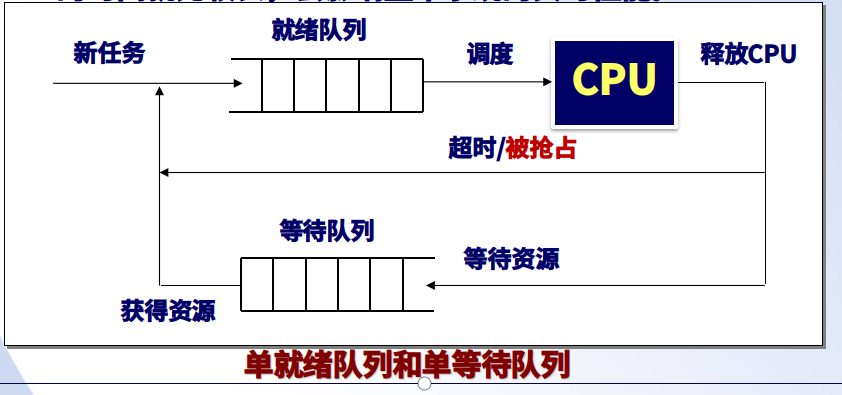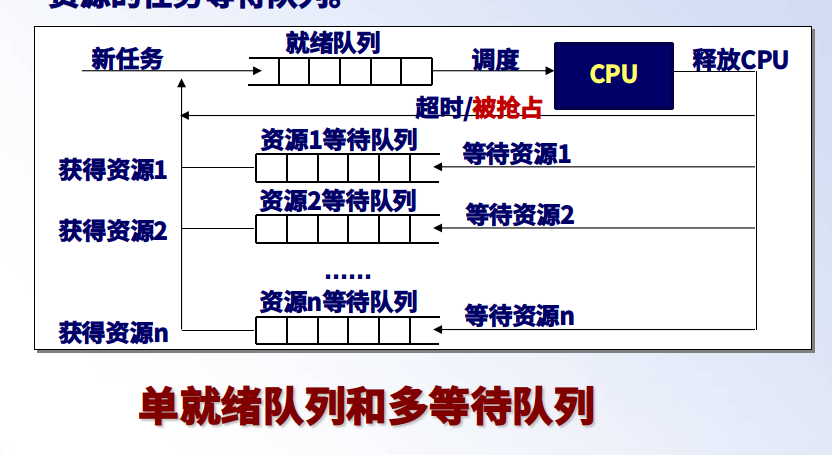• 中断、自陷
• 运行任务因缺乏资源阻塞
• 时间片轮转调度时
• 高优先级任务处于就绪时

• 正在运行的任务可能被其他任务所打断。
• 一旦任务开始运行，该任务只有在运行完成而主动放弃CPU资源，或是因为等待其他资源被阻塞的情况下才会停止运行。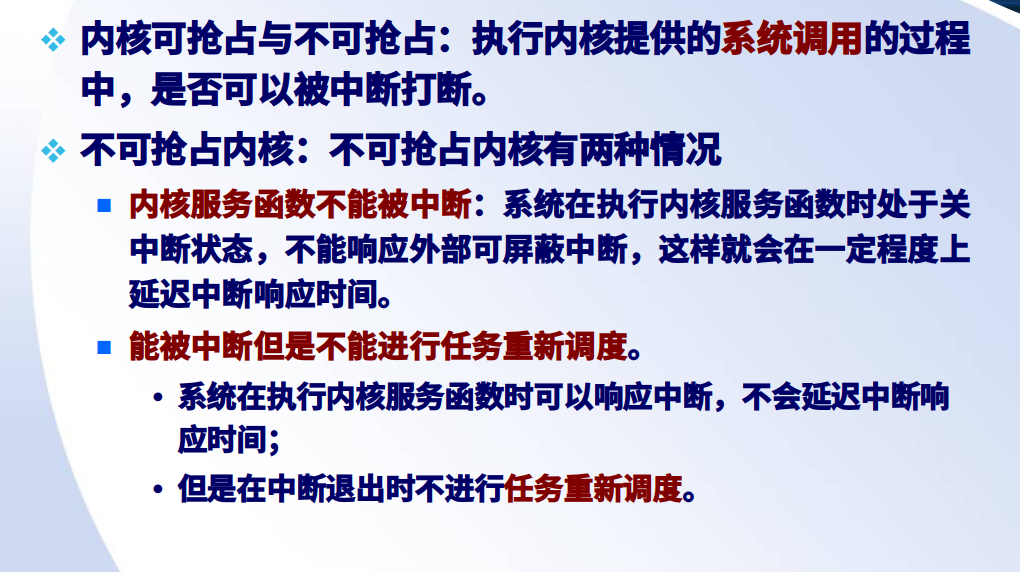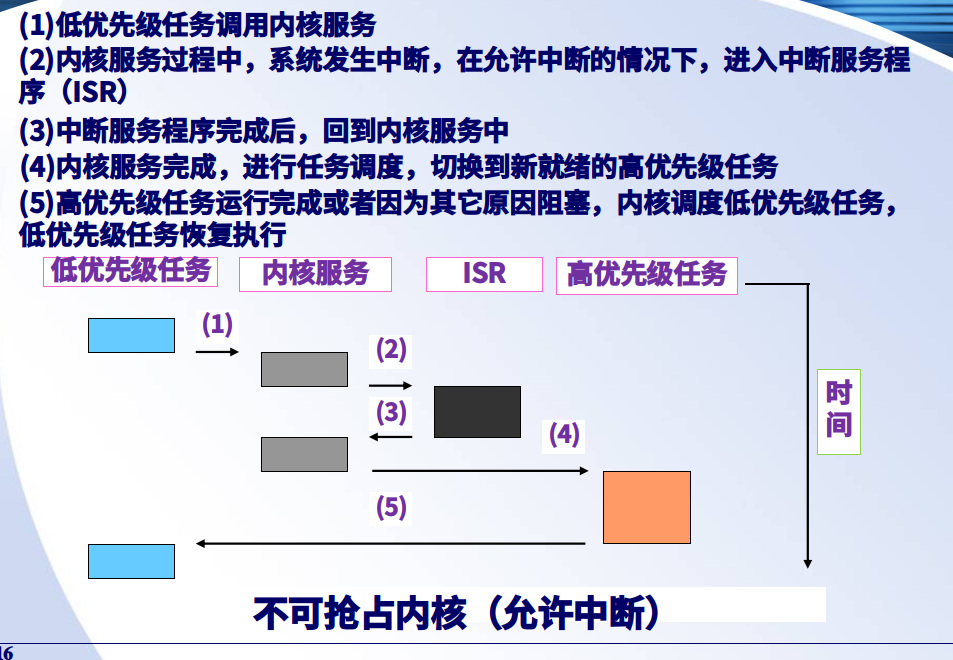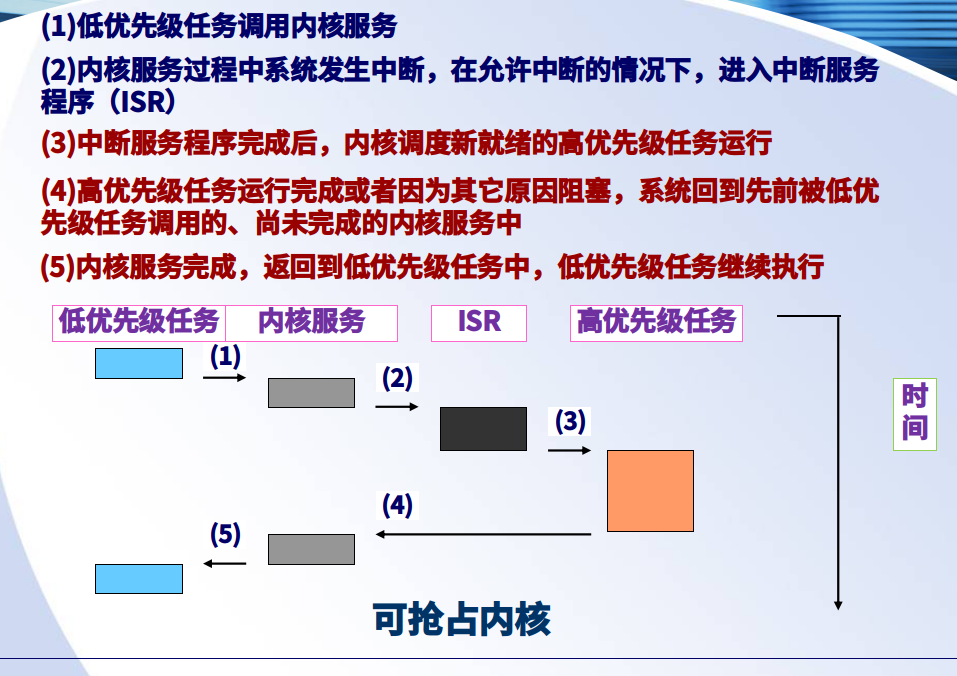• 高优先级任务需要等待低优先级任务释放资源，而低优先级任务又正在等待中等优先级任务的现象。
• 实时操作系统区别于普通操作系统的最主要之一就是“产生结果的时间”

• 优先级继承协议(priority inheritance protocol)
• 优先级天花板协议(priority ceiling protocol)

#include "windows.h"
CRITICAL_SECTION _critical
/*初始化，最先调用的函数。没什么好说的，一般windows编程都有类似初始化的方法*/
InitializeCriticalSection(& _critical)
/*释放资源，确定不使用_critical时调用，一般在程序退出的时候调用。如果以后还要用_critical，则要重新调用InitializeCriticalSection
*/
DeleteCriticalSection(& _critical)
/*

*/
EnterCriticalSection（& _critical）
/*

*/
LeaveCriticalSection(& _critical)

/**
* 创建并启动所有任务
*/
{
INT8U i;
{
}
// Each task will pass its own id
}
/**
* 任务 TA0
*/
{
INT8U err;
INT8U id;
id = *(int *)pdata;
for (;;)
{
printf("Task_%d waitting for an EVENT\n\r", id);
OSTimeDly(200); /* Delay 200 clock tick */
printf("Task_%d trying to GET MUTEX\n\r", id);
OSSemPend(mutex, 0, &err); /* Acquire mutex */
switch (err)
{
case OS_NO_ERR:
break;
default:
printf("Task_%d CANNOT get mutex, then SUSPENDED.\n\r", id);
}
OSTimeDly(200); /* Delay 200 clock tick */
OSSemPost(mutex); /* Release mutex */
}
}
/**
*/
{
INT8U id;
id = *(int *)pdata;
for (;;)
{
printf("Task_%d waitting for an EVEBT\n\r", id);
OSTimeDly(100); /* Delay 100 clock tick */
OSTimeDly(100);
}
}
/*
*/
{
INT8U err;
INT8U id;
id = *(int *)pdata;
for (;;)
{
printf("Task_%d trying to GET MUTEX\n\r", id);
OSSemPend(mutex, 0, &err); /* Acquire mutex */
switch (err)
{
case OS_NO_ERR:
OSTimeDly(200); /* Delay 100 clock tick */
break;
default:
printf("Task_%d CANNOT get mutex, then SUSPENDED.\n\r", id);
OSTimeDly(200); /* Delay 100 clock tick */
break;
}
OSSemPost(mutex); /* Release mutex */
}
}
pdf31页实验指导书

• 概念:是操作系统提供给用户程序调用的一组特殊接口。用户程序可以通过这组特殊接口来获得操作系统内核提供的服务。
• 系统调用并非直接和程序员或系统管理员打交道，它仅仅是一个通过软中断机制向内核提交请求，获取内核服务的接口。
• Linux会有6个寄存器使用来传递这些参数：eax (存放系统调用号)、 ebx、ecx、edx、esi及edi来存放这些额外的参数（以字母递增的顺序）。

#### 信号

#include <signal.h>
#include <stdio.h>
void ouch(int sig){
printf("\nOUCH! - I got signal %d\n", sig);
(void) signal(SIGINT, SIG_DFL);
}
int main(){
(void) signal(SIGINT, ouch);
while(1) {
printf("Hello World!\n");
sleep(1);
}
return 0;
}

#### 示例

server.c
#include<stdio.h>
#include<stdlib.h>
#include<unistd.h>
#include<fcntl.h>
#include<sys/types.h>
#include<sys/ipc.h>
#include<sys/shm.h>
#include<errno.h>
#define SHMSZ 27
int main(){
char c;
int shmid;
key_t key;
char *shm,*s;
key=5678;
shmid=shmget(key,SHMSZ,0666|IPC_CREAT);
if(shmid<0){
printf("shm create error");
exit(1);
}
shm=(char *)shmat(shmid,NULL,0);
if(shm==(char *)-1){
printf("shm at error");
exit(1);
}
s=shm;
for(c='a';c<'z';c++){
*s++=c;
}
*s=NULL;
while(*shm !='*'){
sleep(1);
}
shmdt(shm);
exit(0);
return 0;
}
user.c
#include<stdio.h>
#include<stdlib.h>
#include<unistd.h>
#include<fcntl.h>
#include<sys/types.h>
#include<sys/ipc.h>
#include<sys/shm.h>
#include<errno.h>
#define SHMSZ 27
int main(){
char c;
int shmid;
key_t key;
char *shm,*s;
key=5678;
shmid=shmget(key,SHMSZ,0666|IPC_CREAT);
if(shmid<0){
printf("shm create error");
exit(1);
}
shm=(char *)shmat(shmid,NULL,0);
if(shm==(char *)-1){
printf("shm at error");
exit(1);
}
for(s=shm;*s!=NULL;s++){
putchar(*s);
}
putchar('\n');
*s='*';
shmdt(shm);
exit(0);
return 0;
}

### shell编程题

1.数组排序
c++代码
int nums={1,2,1,3,4,2,5,6};
int length=8;
for(int i=0;i<length;i++){
for(int j=i+1;j<length;j++){
if(nums[j]<nums[i]){
int t=nums[j];
nums[j]=nums[i];
nums[i]=t;
}
}
}
for(int i=0;i<length;i++){
cout<<nums[i]<<" ";
}
shell代码
i=0 #外层循环计数
j=0+\$i #内层循环计数
t=0 #用于两数字交换临时变量
nums=(1 2 1 3 4 2 5 6) # 用于测试的数组
length=\${#nums[*]} # 数组长度
# 外层循环开始
while [ \$i -lt \$length ]
do
# 内层循环开始
j=\$((\$i + 1))
while [ \$j -lt \$length ]
do
# 从小到大排序
if [ \${nums[\$j]} -lt \${nums[\$i]} ]
then
t=\${nums[\$i]}
nums[\$i]=\${nums[\$j]}
nums[\$j]=\$t
fi
# j++
j=\$((\$j + 1))
done
# i++
i=\$((\$i + 1))
done
# 输出结果
i=0
while [ \$i -lt \$length ]
do
echo -e "\${nums[\$i]} \c"
# i++
i=\$((\$i + 1))
done
2.数组求和
nums=(3 2 3 4 5)
sum=0
for v in \${nums[@]}
do
sum=\$((\$sum + \$v))
done
echo \$sum
3.求最值
max=\${nums}
for v in \${nums[@]}
do
if [ \$max -lt \$v ]
then
max=\$v
fi
done
echo \$max
4.水仙花数(100-999).水仙花数是指一个 3 位数，它的每个位上的数字的 3次幂之和等于它本身
c++代码
int i=100;
while(i<=999){
int sum=0;
int temp=i;
int k=0;
while(temp!=0){
k=temp%10;
sum=sum+k*k*k;
temp=temp/10;
}
if(sum==i){
cout<<sum<<" ";
}
i++;
}
shell 代码
# @author sugar
# time 2020年 01月 01日 星期三 22:33:59 CST
# 水仙花数
i=100
# 外层循环遍历每个数字(100 - 999)
while [ \$i -le 999 ]
do
declare -i sum=0 #存放3个位数和的临时值
declare -i temp=\$i #当前待判断的值
declare -i k=0 #临时存放每个位数的值
while [ temp -ne 0 ]
do
k=\$((\$temp % 10))
temp=\$((\$temp/10))
sum=\$((\$sum+\$k*\$k*\$k))
done
# 如果相等即为水仙花数
if [ \$sum -eq \$i ]
then
echo -e "\$sum \c"
fi
# i++
i=\$((\$i + 1))
done